# Maximum Element in a Linked List

## Finding Maximum Element in a Linked List using C Language:

In this article, I am going to discuss How to Find the Maximum Element in a Linked List using C Language with Examples. Please read our previous article, where we discussed the Sum of all elements in a Linked List using C Language with Examples.

##### How to Find the Maximum Element in a Linked List

So first we will show you it’s working then we will write on a function for it.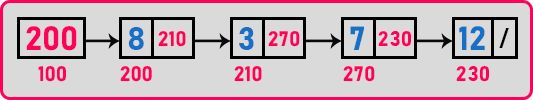We want to find out the maximum element so we have to traverse all these elements by taking a pointer and letting the pointer point to all the elements of a given linked list.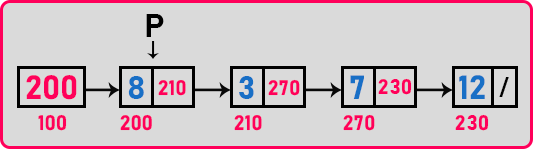For storing the maximum element, we should have some variable ‘max’. Then it should have some initial value. This Max should have some initial value to compare with some other number. it should have some small number so that the other number becomes larger than this number. So what should be the initial value?

We should have some smaller number so,

int max = MIN_INT;

Here we are string smallest integer in ‘max’ variable. So, we will assume that the smallest integer is -32768. It is 16 bits integer that is 2 bytes integer. This is the smallest number we can write. Any value other than ‘-32768’ will definitely be greater because this is the smallest one. Now let us see the procedure.

##### Procedure for finding the max element in a linked list: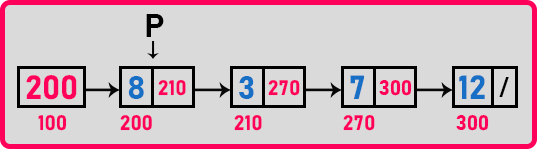Here ‘p’ is pointing to the node which has the address ‘200’ and data ‘8’. So, we will check for if (max < p->data) i.e. -32768 < 8. So we will modify max as ‘max = p->data’. Now max is ‘8’.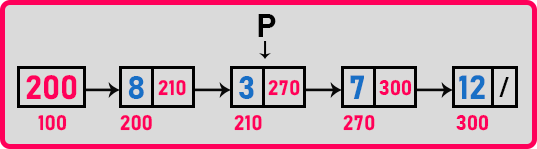Now, ‘p’ is pointing on the next node of address ‘210’ and data ‘3’. Here also we will check for if (max < p->data) i.e. 8 < 3? No, so we will not change max.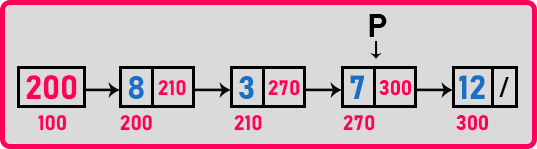Now, ‘p’ is pointing on the next node of address ‘270’ and data ‘7’. Here also we will check for if (max < p->data) i.e. 8 < 7? No, so we will not change max.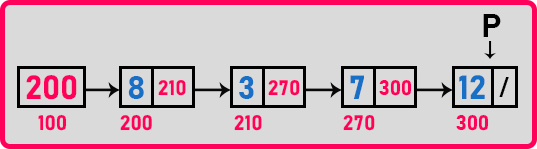Here ‘p’ is pointing on the node which has the address ‘300’ and data ‘12’. So, we will check for if (max < p->data) i.e. 8 < 12? Yes, so we will modify max as ‘max = p->data’. Now max is ‘12’. Now the linked list is completed and we get the maximum element which is ‘12’. Now let us write the function.

##### Function to find max element in a linked list:
###### Iterative function:
```int max(Node * p)
{
int m = -32768;
while (p)
{
if (p->data > m)
m = p->data;
p = p->next;
}
return (m);
}
```
###### Recursive Function:
```int Rmax(Node * p)
{
int x = 0;
if (p == 0)
return MIN_INT;
else
{
x = Rmax (p->next);
if (x > p->data)
return x;
else
return p->data;
}
}
```

We can rewrite the same recursive function in a different way. Now we will show you.

```int Rmax(Node * p)
{
int x = 0;
if (p == 0)
return MIN_INT;
x = Rmax (p->next);
return x > p - data ? x : p->data;
}
```

So here we have used Ternary Operator. So, this is a function for finding the maximum element in the linked list Let us see the full program:

##### Program to find max element in a linked list using C Language:
```#include <stdio.h>
#include <stdlib.h>
#include <limits.h>
struct Node
{
int data;
struct Node *next;
}   *first = NULL;

void Create(int A[], int n)
{
int i;
struct Node *t, *last;
first = (struct Node *) malloc(sizeof (struct Node));
first->data = A;
first->next = NULL;
last = first;

for (i = 1; i < n; i++)
{
t = (struct Node *) malloc(sizeof(struct Node));
t->data = A[i];
t->next = NULL;
last->next = t;
last = t;
}
}

int Max(struct Node *p)
{
int max = INT_MIN;

while (p)
{
if (p->data > max)
max = p->data;
p = p->next;
}
return max;
}

int RMax(struct Node *p)
{
int x = 0;
if (p == 0)
return INT_MIN;
x = RMax (p->next);
if (x > p->data)
return x;
else
return p->data;
}

int main()
{
int A[] = { 8, 3, 7, 12 };
Create(A, 4);
printf ("Max %d\n", Max (first));
return 0;
}
```
###### Output: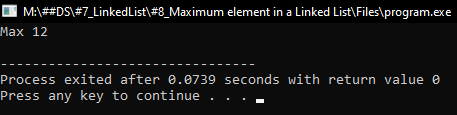In the next article, I am going to discuss Linear Search in a Linked List using C Language with Examples. Here, in this article, I try to explain Finding Maximum Element in a Linked List using C Language with Examples and I hope you enjoy this How to Find Max Element in a Linked List using C Language with Examples article.